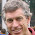## Monday, December 28, 2009

### ParaSail character, string, and numeric literals

Almost all programming languages these days have special syntax for character literals (generally using single quotes), string literals (generally using double quotes), and numeric literals (digits, plus a decimal point for floating point, and optionally some sort of radix indicator for octal, hex, etc.).  Languages differ in how they distinguish among multiple string, character, or numeric types in the syntax for literals.  ParaSail adopts the usual syntax for these literals, but treats them each as being of a particular universal type.  Other "normal" types can provide conversions to and from these universal types, and thereby gain use of the corresponding literal notation.

The four basic kinds of literals in ParaSail, and their corresponding universal types, are as follows:

kind of literal
example
universal type
string literal
"this is a string literal"
Univ_String
character literal
'c'
Univ_Character
integer literal
42
Univ_Integer
real literal
3.14159
Univ_Real

The universal types can be used at run-time, but they are primarily intended for use with literals and in annotations.  Univ_String corresponds to UTF-32, which is a sequence of 32-bit characters based on the ISO-10646/Unicode standard.  Univ_Character corresponds to a single 32-bit ISO-10646/Unicode character (actually, only 31 bits are used).  Univ_Integer is an "infinite" precision signed integer type.  Univ_Real is an "infinite" precision signed rational type, with signed zeroes and signed infinities.

The universal numeric types have the normal four arithmetic operators, "+", "-", "*", "/".  They both also have an exponentiation operator "**", with signed Univ_Integer exponents for Univ_Real and non-negative Univ_Integer exponents for Univ_Integer.  Univ_Integer also has "mod" and "rem", corresponding to remainder operations, with "rem" being the remainder for "normal" truncate-toward-zero division, and "mod" being the remainder for truncate-toward-negative-infinity division.

Bitwise operators "and", "or", and "xor" are defined for non-negative Univ_Integers.  "<<" and ">>" are for shifting Univ_Integers, with "<<" defined as equivalent to multiplying by the corresponding power of two, and ">>" defined as equivalent to dividing by the corresponding power of two, but with truncation toward negative infinity.

By providing conversions to and from a universal type, a "normal" type can support the use of the corresponding literal.  These special conversion operations are declared as follows (these provide for integer literals):
```operator "from_univ"(Univ : Univ_Integer)
-> My_Integer_Type;
operator "to_univ"(Int : My_Integer_Type)
-> Univ_Integer;
```
If an interface provides the operator "from_univ" converting from a given universal type to the type defined by the interface, then the corresponding literal is effectively overloaded on that type.  The complementary operator "to_univ" is optional, but is useful in annotations to connect operations on a user-defined type back to the predefined operators on the universal types.

Annotations may be provided on the conversion operators to indicate the range of values that the conversion operators accept. So for a 32-bit integer type we might see the following:
```interface Integer_32<> is
operator "from_univ"
(Univ : Univ_Integer {Univ in -2**31 .. +2**31-1})
-> Integer_32;
operator "to_univ"(Int : Integer_32)
-> Result: Univ_Integer {Result in -2**31 .. +2**31-1};
...
end interface Integer_32;
```
With these annotations it would be an error to write an integer literal in a context expecting an Integer_32 if it were outside the specified range.

1.2.